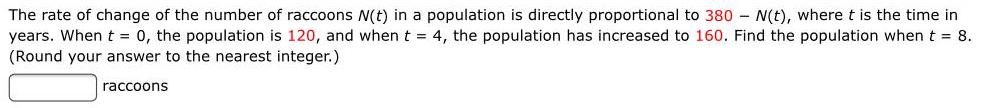Question:

# The rate of change of the number of raccoons N(t) in a

Last updated: 8/8/2022The rate of change of the number of raccoons N(t) in a population is directly proportional to 380 N(t), where t is the time in years. When t = 0, the population is 120, and when t = 4, the population has increased to 160. Find the population when t = 8. (Round your answer to the nearest integer.) raccoons